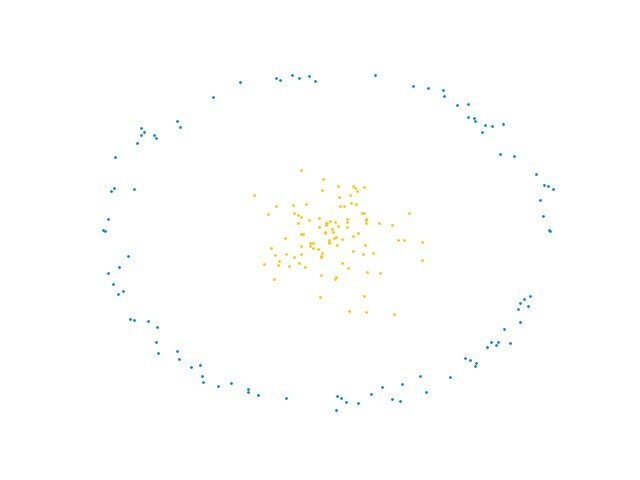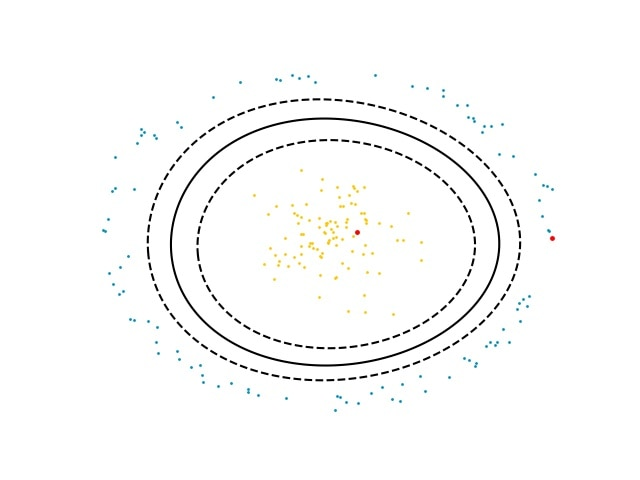## Train an SVM classifier with scikit-learn

In this section of the guide, we focus on how to train an SVM classifier with scikit-learn and how to dump the parameters for use with CMSIS-DSP. The data generation and visualization parts of this activity are beyond the scope of this guide.

The code below can be found in the CMSIS-DSP library:
CMSIS/DSP/Examples/ARM/arm_svm_example/train.py

You can run this example to reproduce the results of this guide, so that you can generate the data, train the classifier and display some pictures.

Let's look at the parts of this script that correspond to the training and dumping of parameters.

The training of the SVM classifier relies on the scikit-learn library. So, we must import svm from the sklearn module.

Training requires some data. The random, numpy, and math Python modules are imported for the data generation part . More modules are required for the graphic visualization. This is described in the train.py file.

The following Python code loads the required modules:

```from sklearn import svm
import random
import numpy as np
import math```

The data is made of two clusters of 100 points. The first cluster is a ball that is centered around the origin. The second cluster has the shape of an annulus around the origin and the previous ball.

This image shows what those clusters of points look like:Figure 3: Two clusters of points that are not linearly separable

The cluster of points were generated with the following Python code. This code generates the random points and prepares the data for the training of the classifier. The data is an array of points and an array of corresponding classes: X_train and Y_train

The yellow points correspond to class 0 and the blue points correspond to class 1.

```NBVECS = 100
VECDIM = 2

x = ballRadius * np.random.randn(NBVECS, 2)

angle = 2.0 * math.pi * np.random.randn(1, NBVECS)
radius = 3.0 + 0.1 * np.random.randn(1, NBVECS)

xa = np.zeros((NBVECS,2))
xa[:, 0] = radius * np.cos(angle)
xa[:, 1] = radius * np.sin(angle)

X_train = np.concatenate((x, xa))
Y_train = np.concatenate((np.zeros(NBVECS), np.ones(NBVECS)))```

The following two lines create and train a polynomial SVM classifier using the data that we just defined:

`clf = svm.SVC(kernel='poly', gamma='auto', coef0=1.1)clf.fit(X_train, Y_train)`

You can see the result of the training in the following image:Figure 4: Polynomial SVM frontier separating two clusters of points

The solid line represents the separation between the two classes, as the SVM classifier learned.

The larger red points on the image are two test points that are used to check the classifier.

The red point near the center of the image is inside class 0. The red point near the edge of the image corresponds to class 1.

The following code creates the first point inside the center cluster, the class 0, and applies the classifier. The result of predicted1 should be 0:

```test1 = np.array([0.4,0.1])
test1 = test1.reshape(1,-1)

predicted1 = clf.predict(test1)
print(predicted1)```

Now, we would like to use this trained classifier with the CMSIS-DSP. For this, the parameters of the classifier must be dumped.

The CMSIS-DSP polynomial SVM uses the instance structure that is shown in the following code. The parameters of this structure are needed by CMSIS-DSP and must be dumped from the Python script:

```typedef struct
{
uint32_t        nbOfSupportVectors;     /**< Number of support vectors */
uint32_t        vectorDimension;        /**< Dimension of vector space */
float32_t       intercept;              /**< Intercept */
const float32_t *dualCoefficients;      /**< Dual coefficients */
const float32_t *supportVectors;        /**< Support vectors */
const int32_t   *classes;               /**< The two SVM classes */
int32_t         degree;                 /**< Polynomial degree */
float32_t       coef0;                  /**< Polynomial constant */
float32_t       gamma;                  /**< Gamma factor */
} arm_svm_polynomial_instance_f32;```

Other SVM classifiers, for example linear, sigmoid, and rbf, are used in a similar way but require fewer parameters than the polynomial one. This means that, as soon as you know how to dump parameters for the polynomial SVM, you can do the same for other kinds of SVM classifiers.

The following Python script accesses the parameters from the trained SVM classifier and prints the values for use in CMSIS-DSP:

```supportShape = clf.support_vectors_.shape

nbSupportVectors = supportShape
vectorDimensions = supportShape

print("nbSupportVectors = %d" % nbSupportVectors)
print("vectorDimensions = %d" % vectorDimensions)
print("degree = %d" % clf.degree)
print("coef0 = %f" % clf.coef0)
print("gamma = %f" % clf._gamma)

print("intercept = %f" % clf.intercept_)```

Support vectors and dual coefficients are arrays in CMSIS-DSP. They can be printed with the following code:

```dualCoefs = clf.dual_coef_
dualCoefs = dualCoefs.reshape(nbSupportVectors)
supportVectors = clf.support_vectors_
supportVectors = supportVectors.reshape(nbSupportVectors * VECDIM)

print("Dual Coefs")
print(dualCoefs)

print("Support Vectors")
print(supportVectors)```